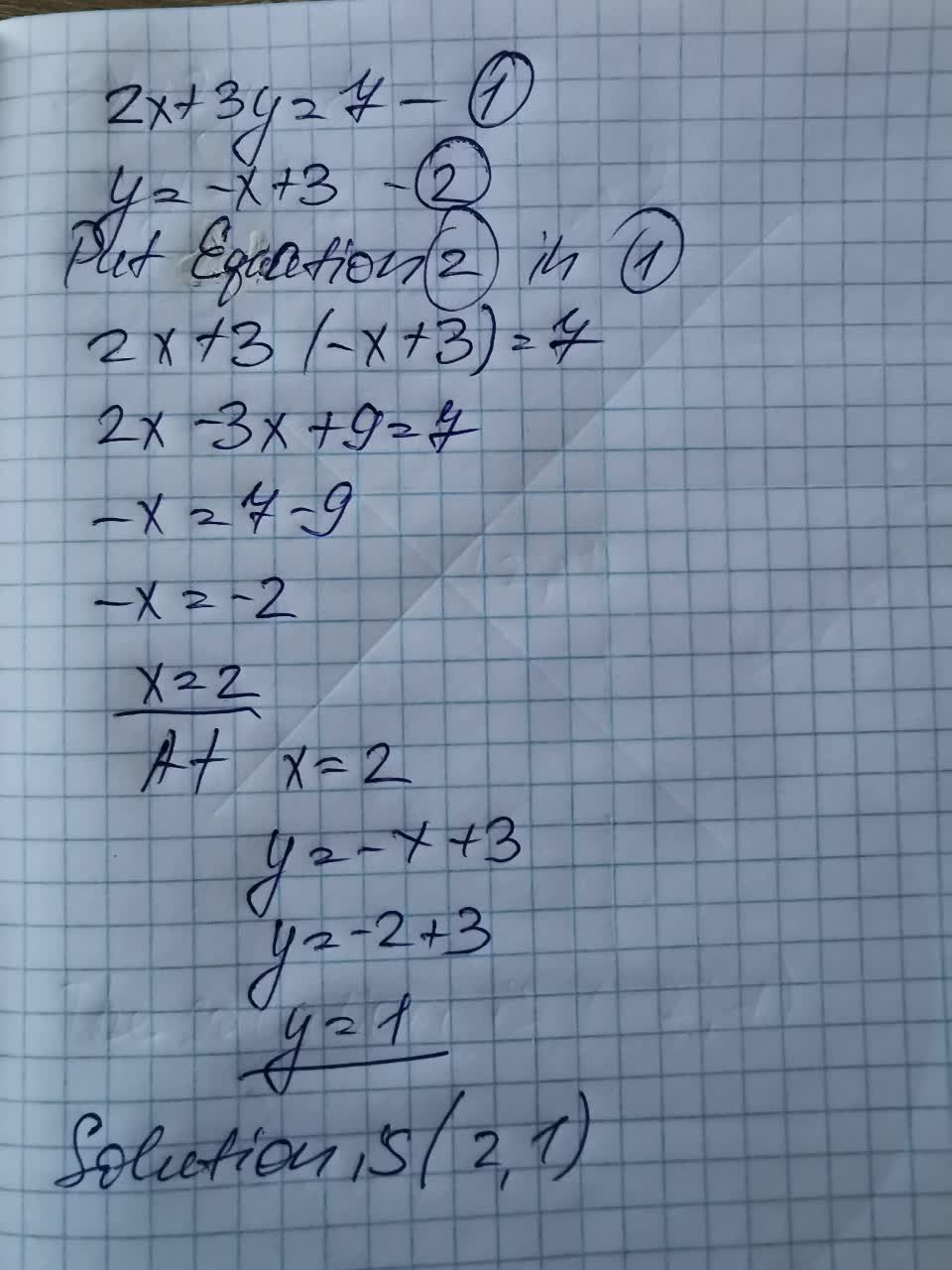# What is the solution to the systems of equations below? 2x + 3y = 7 , y =-x + 3naivlingr 2021-09-15 Answered

What is the solution to the systems of equations below? $\left\{\begin{array}{l}2x+3y=7\\ y=-x+3\end{array}$

You can still ask an expert for help

• Questions are typically answered in as fast as 30 minutes

Solve your problem for the price of one coffee

• Math expert for every subject
• Pay only if we can solve itIsma Jimenez